HECKSCHEROHLIN THEORY p What determines comparative advantage p

• Slides: 16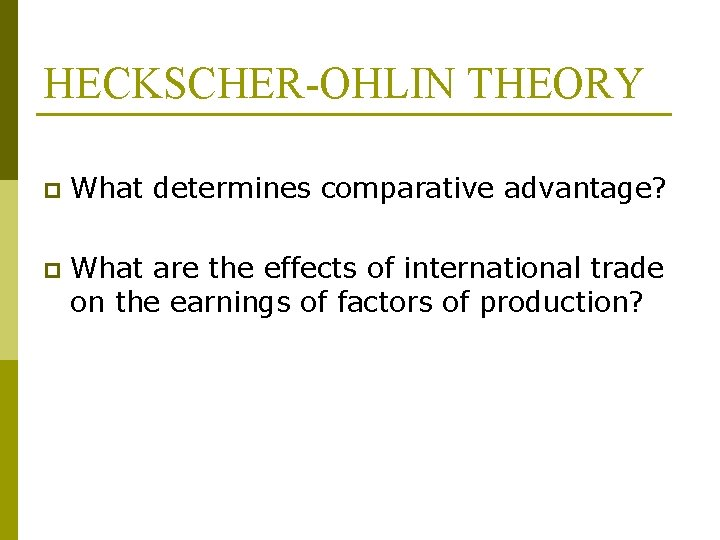HECKSCHER-OHLIN THEORY p What determines comparative advantage? p What are the effects of international trade on the earnings of factors of production?HECKSCHER-OHLIN THEORY p Assumptions: 1. There are 2 nations (Nation 1 and Nation 2), 2 commodities (Commodity X and Y), and 2 factors of production (Capital and Labor). 2. Both nations use the same technology in production. 3. Commodity X is labor intensive and commodity Y is capital intensive in both nations p Commodity X requires relatively more labor to produce than commodity Y. p (K/L)X < (K/L)Y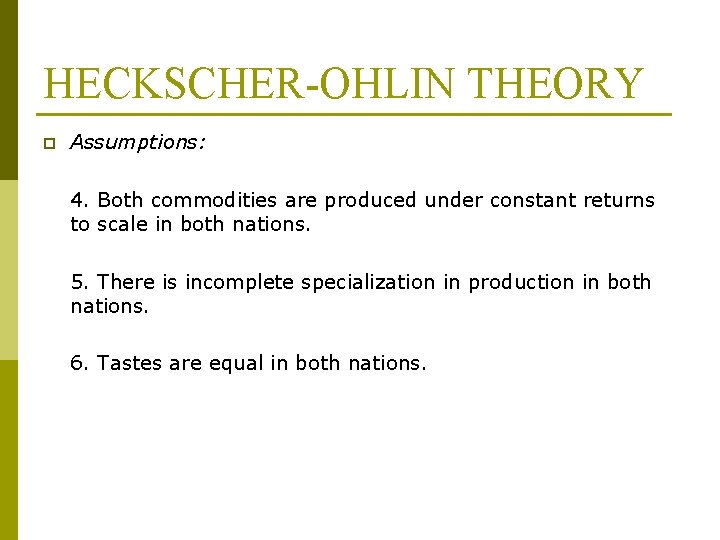HECKSCHER-OHLIN THEORY p Assumptions: 4. Both commodities are produced under constant returns to scale in both nations. 5. There is incomplete specialization in production in both nations. 6. Tastes are equal in both nations.HECKSCHER-OHLIN THEORY p Assumptions: 7. There is perfect competition in both commodities and factor markets in both nations. p p Producers and consumers are too small to affect the price level. Normal profit Homogeneous products and inputs. Perfect knowledge. 8. There is perfect factor mobility within each nation but no international factor mobility.HECKSCHER-OHLIN THEORY p Assumptions: 9. There are no transportation costs, tariffs or other obstructions to the free flow of international trade. 10. All resources are fully employed in both nations. 11. International trade between two nations is balanced.HECKSCHER-OHLIN THEORY p Factor Intensity: n Commodity Y is capital intensive if the (K/L) ratio used in the production Y is greater than (K/L) used in the production of X. n Commodity X is labor intensive if the (K/L) ratio used in the production X is smaller than (K/L) used in the production of Y.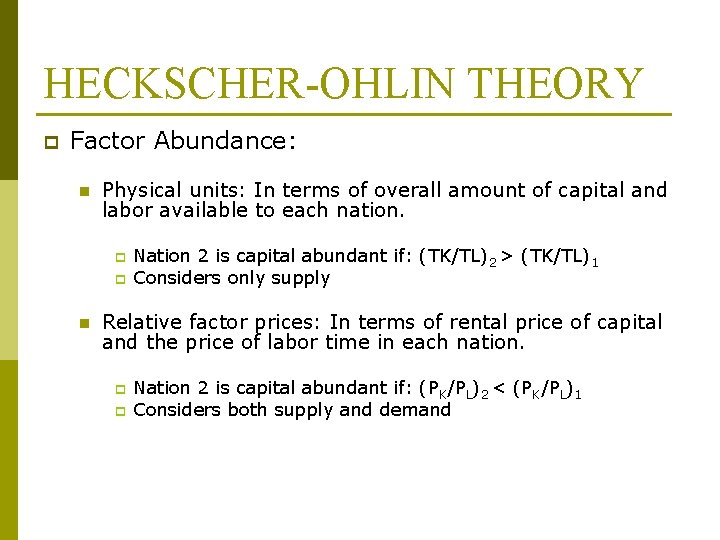HECKSCHER-OHLIN THEORY p Factor Abundance: n Physical units: In terms of overall amount of capital and labor available to each nation. p p n Nation 2 is capital abundant if: (TK/TL)2 > (TK/TL)1 Considers only supply Relative factor prices: In terms of rental price of capital and the price of labor time in each nation. p p Nation 2 is capital abundant if: (PK/PL)2 < (PK/PL)1 Considers both supply and demandHECKSCHER-OHLIN THEORY p Factor Abundance: With (TK/TL) larger in Nation 2 than in Nation 1 in the face of equal demand conditions (and technology), (PK/PL) will be smaller in Nation 2. Thus Nation 2 is the K-abundant nation in terms of both definitions.HECKSCHER-OHLIN THEORY p Heckscher-Ohlin Theorem: A nation will export the commodity whose production requires the intensive use of the nation’s relatively abundant and cheap factor and import the commodity whose production requires the intensive use of the nation’s relatively scarce and expensive factor.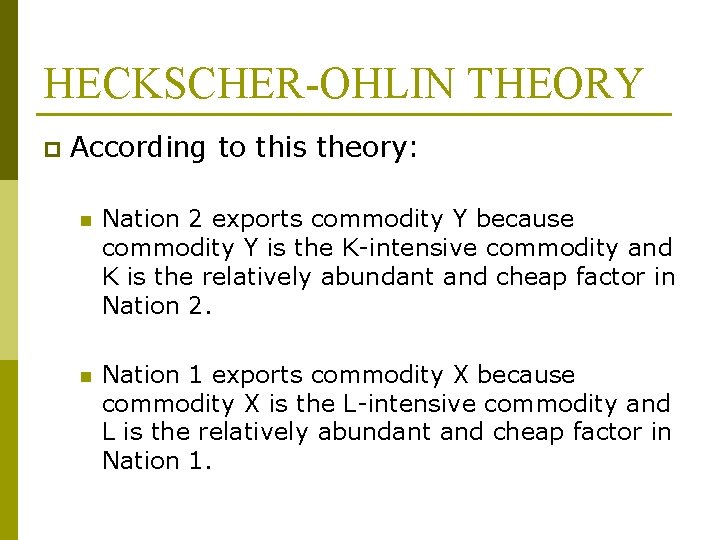HECKSCHER-OHLIN THEORY p According to this theory: n Nation 2 exports commodity Y because commodity Y is the K-intensive commodity and K is the relatively abundant and cheap factor in Nation 2. n Nation 1 exports commodity X because commodity X is the L-intensive commodity and L is the relatively abundant and cheap factor in Nation 1.HECKSCHER-OHLIN THEORY p H-O Theorem isolates the difference in relative factor abundance among nations as the basic cause or determinant of comparative advantage. So, this theory is also called FACTOR PROPORTIONS or FACTOR-ENDOWMENT THEORY.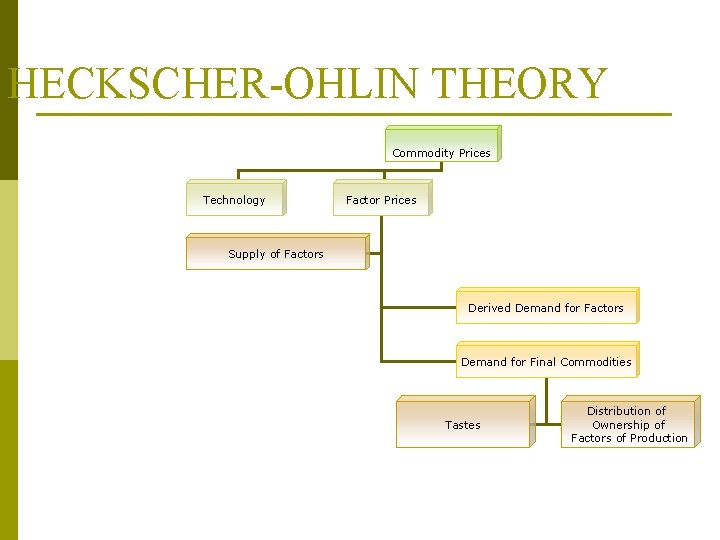HECKSCHER-OHLIN THEORY Commodity Prices Technology Factor Prices Supply of Factors Derived Demand for Factors Demand for Final Commodities Tastes Distribution of Ownership of Factors of Production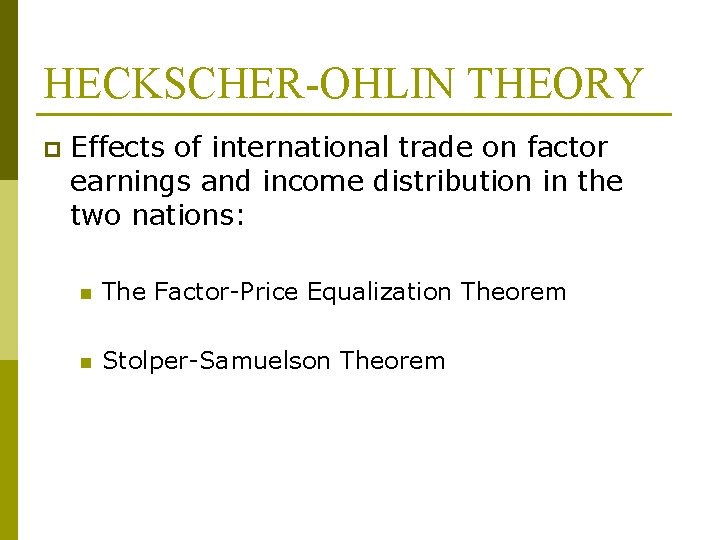HECKSCHER-OHLIN THEORY p Effects of international trade on factor earnings and income distribution in the two nations: n The Factor-Price Equalization Theorem n Stolper-Samuelson TheoremHECKSCHER-OHLIN THEORY p The Factor-Price Equalization Theorem: n H-O-S Theorem (Paul Samuelson) n Analysis the effect of international trade on the difference in factor prices between nations. n International trade will bring about equalization in the relative and absolute returns to homogeneous factors across nations. p p Wages of homogeneous labor will be same in all trading nations. Return to homogeneous capital will be same in all trading nations.HECKSCHER-OHLIN THEORY p Stolper-Samuelson Theorem: n Analysis the effects of international trade on relative factor prices and income within each nation. n Trade increases the price of the nation’s abundant and cheap factor, and reduces the price of its scarce and expensive factor. p p Nation 1 (labor abundant): The real income of labor rises, and the real income of capital falls. Nation 2 (capital abundant): The real income of labor falls, and the real income of capital rises.Empirical Tests of the H-O Theorem p W. Leontief (1951) p Leontief Paradox: U. S. import substitutes were 30% more K -intensive than U. S. exports. So, U. S. imports K-intensive commodities and exports L-intensive commodities. n US labor is more productive than foreign labor p Explanations of the Leontief Paradox: n The year 1947 is not representative n Other factors of production like natural resources are not considered. n US tariff policy protects L-intensive industries n Ignored human capital (education, job training etc. ) p H-O Theorem is useful in explaining trade in raw materials, agricultural products and labor-intensive manufactures, between developing and developed nations.